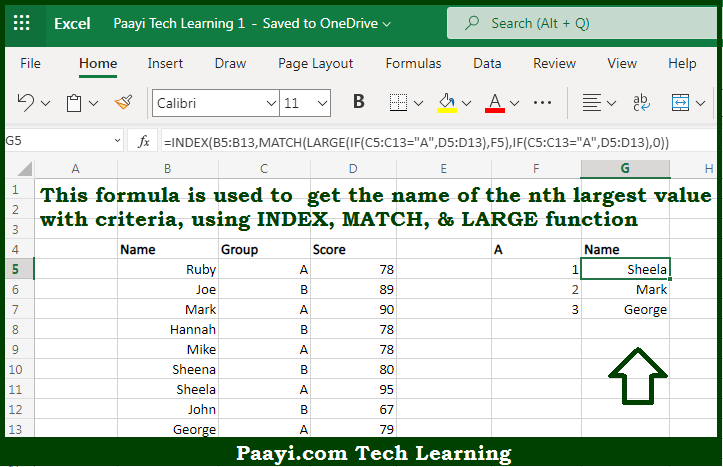# Learn How to Name nth Largest Value With Criteria in Microsoft Excel

Written by | 0 Comments | 354 Views

In this article, you will learn how to evaluate things with MIN and MAX functions in Microsoft Excel using a single/combination(s) of functions. You will also know to Name nth Largest Value With Criteria and see the generic formula.

Learn How to Name nth Largest Value With Criteria in Microsoft Excel

The main purpose of this formula is to get the name of the nth largest value with criteria. Here we will learn how to name the nth largest value when criteria are given in the given data range in the workbook in Microsoft Excel. That implies, with the help of a formula based on the INDEX, MATCH, and LARGE functions you can able to get the name of the nth largest value with criteria. So, with the help of this formula, you can able to name the nth largest value when criteria are given in the given data range in the workbook in Microsoft Excel.

General Formula to Name nth Largest Value

=INDEX(range,MATCH(LARGE(filtered_range,F5),filtered_range,0))

The Explanation to Name nth Largest ValueSo we know that with the help of the given formula above you can able to get the name of the nth largest value with criteria. Here we will learn how to name the nth largest value when criteria is given in the given data range in the workbook in Microsoft Excel. As we know that this formula utilizes the LARGE function to find the nth largest value in the data set. Applying criteria to the IF function, which is used to "filter" values before they are evaluated with LARGE. while working from the inside out, the initial step is to get the "1st" largest value in the data range associated with Group A with the LARGE function. So now you have learned how to name the nth largest value when criteria is given in the given data range in the workbook in Microsoft Excel.